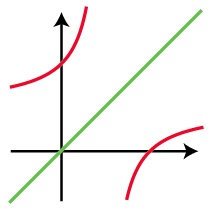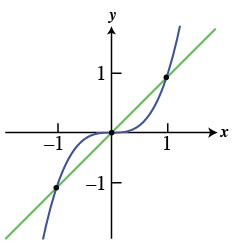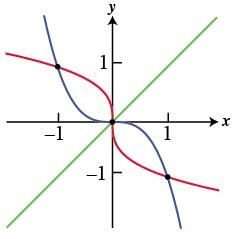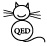# There’s madness in the Methods

by Burkard Polster and Marty Ross

The Age, 23 April 2012In its first year in 2007, this column appeared in VCE Express, a section of The Age devoted to helping VCE students and teachers. However, we never actually wrote about VCE mathematics. We had decided that there was no point.

At the time, we simply couldn’t see any interesting way to write about VCE maths. That judgment was possibly too dismissive of the subject Specialist Mathematics. Unfortunately, in regard to Mathematical Methods, we are more convinced than ever.

As an example of the problem, consider the number e, which we wrote about last year. We hope what we wrote was interesting and we plan to write more. However, interesting or not, we don’t believe that anything we might write on e could help a VCE student do well in their assessment.

The number e is introduced in the year 12 subject Mathematical Methods 3 & 4, but is simply treated as a mystery number. A Methods student need know nothing more than that e has magical calculus properties, and that there’s a button for it somewhere on the calculator. Actually knowing what e is, or why it has the properties that it does, seems to be of no consequence.

How did Methods get this way? As exemplified by the treatment of e, those who have mandated the huge emphasis on calculators have plenty to answer for. However, the current technology fetishism is merely a symptom of a much more general problem.

Once upon a time, Victorian year 12 students could take Pure Mathematics and Applied Mathematics. They were strong subjects, and the natural division of topics meant that both subjects were well structured and had a clear purpose.

At some point, someone made the very regrettable decision to alter this natural structure. As a consequence, we are now burdened with Methods, a fish-fowl subject devoted to nothing in particular.

True, there are the “methods”. However, these methods are often contrived or have little purpose. Moreover, as we’ll see, they’re sometimes plain wrong.

The technical nature of Methods makes it difficult to indicate the subject’s deep and systemic problems. Those familiar with the subject may be interested in a textbook review we have written in collaboration with our colleague David Treeby. Here, we’ll attempt to give some sense of the problems by considering aspects of Methods’ treatment of the topic of functions.

Functions are fundamental to mathematics and its applications. They offer a natural way of describing physical processes, of obtaining outputs from inputs. For example, if we have a cooling cup of coffee, we can describe the temperature (output) of the coffee as a function of the time (input) it has been standing.

Functions can also be purely abstract. The cubing function, for example, spits out the cube of whatever number we put in: an input of 2 results in an output of 23 = 8, and so on. Using the standard notation of x for the input and y for the output, we can write the cubing function as y =x3. We can also graph the function using the familiar Cartesian coordinates.Though functions are very natural, there are some subtle notions in the precise mathematical definitions. A solid pure mathematics subject would deal with these subtleties, though one can often get by with intuition alone. What should be avoided is a meaningless middle ground, and engaging in sporadic pedantry, without regard for purpose or clarity: that is the approach taken in Methods.

Methods introduces confusing and pointless technicalities into determining when the composition of functions makes sense (Composition is the operation of following one process by another). If we take the subject guidelines (page 130) literally, then Question 4 on is actually concerned with a function that does not exist. This is absurd, but there is worse to come.

A fundamental method of attempting to understand functions is through the concept of the inverse. The idea is to reverse the process of a function, interchanging the roles of input and output. For our cooling cup of coffee, the inverse function would amount to considering the time as a function of the temperature. Similarly, we can consider our cubing function; its inverse is the cube root function, y = 3x. So, putting in 8 gives an output of 3√8 = 2, and so on.

Actually, there’s a hidden trickiness to inverses, a very important trickiness that is never even addressed in Methods. However, we’ll leave that discussion for another day, and for now we’ll accept such inverse functions at face value.

We now consider two questions that a Methods student might be asked about a function.

The first question: when are the input and the output of a function equal? Notice that this can be a very artificial question: for our coffee cup example, it would amount to asking when the time and the temperature are the same, which is meaningless.

However, though Methods gives no hint of it, there are natural contexts for this question. In any case, purely as an abstract question, it can help us understand the way functions work.

For the cubing function, this question amounts to asking when x3 equals x: it is not difficult to check that these values of x are exactly -1, 0 and 1. Note that the corresponding points on the graph occur on the straight line y = x, and it is easy to see that the same is true for any function.Now for the second question: when are the outputs of a function and its inverse the same?

This appears to be a more difficult question: for our cubic function it amounts to asking when x3 equals 3x. However, we can check that the solutions are again -1, 0 and 1. Moreover, it is not difficult to see that for any function, a solution to the first question will always be a solution to the second question as well.So, maybe the two questions amount to the same problem? If one believes the on Question 2.3 (where the function 3 – 2x3 is considered), this is indeed the case.

Unfortunately, what is written in the 2011 Report (and similarly in the Report on Question 2.1 of the 2010 Exam) is utter nonsense.

It is simply not the case that that the two questions are interchangeable: the solutions to the first question give no insight into whether there are further solutions to the second question, or what they might be. Moreover, the second question is in general much harder to solve than the first.

To give one example out of zillions, consider the function –x3. For this function the only solution to the first question is 0. However, the second question has additional solutions -1 and 1.So, what about 2012? If such a question appears again, should a student go to the trouble of solving it in a mathematically valid manner? Or, should they simply do the quicker nonsense that is expected? We have no idea. Except, that to even have to ask such a question is indicative of a culture of mathematical madness.

Puzzle to Ponder: Consider the function y = (x - 2)/(x - 1). When are the input and the output of this function the same? When are the outputs of this function and its inverse the same?

Burkard Polster teaches mathematics at Monash and is the university's resident mathemagician, mathematical juggler, origami expert, bubble-master, shoelace charmer, and Count von Count impersonator.

Marty Ross is a mathematical nomad. His hobby is smashing calculators with a hammer.

www.qedcat.com

Copyright 2004-∞All rights reserved.